### Home > A2C > Chapter 11 > Lesson 11.2.4 > Problem11-94

11-94.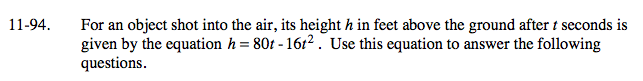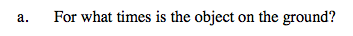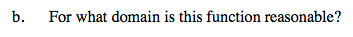Substitute 0 for h.

80t − 16t2 = 0

It is on the ground at 0 and 5 seconds.

Factor the equation.

16t(5 − t) = 0

When is the height positive?

D: 0 ≤ t ≤ 5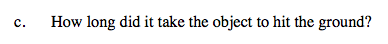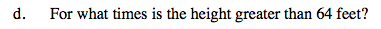At what time will the object be on the ground, not including when it starts?

5 seconds

16t 2 − 80t + 64 = 0

Substitute 64 for h and solve for t.

Factor the equation.

(t − 1)(t − 4)

Simplify.

t 2 − 5t + 4

t = 1, 4

Solve for t.

Use these values to find height greater that 64 ft.

1< t < 4